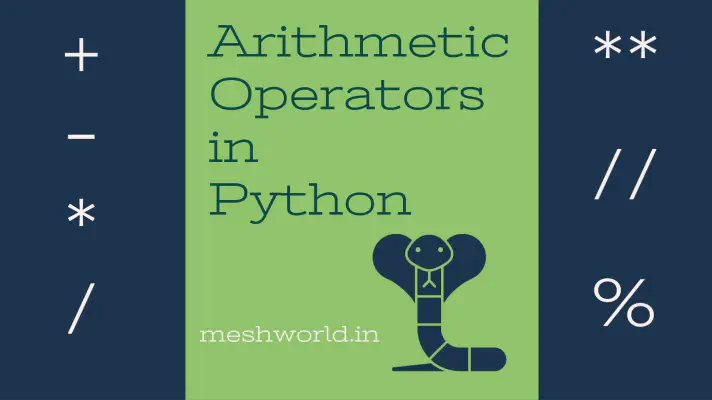# Python - Arithmetic OperatorsPython supports different arithmetic operators that can be used to performs mathematical calculations on the values and variables.

The basic arithmetic operations include addition, subtraction, multiplication, division.

Arithmetic operations are performed according to the order or precedence of operators.

# The general structure of every expression

Every expression will follow this general structure

Operand Operator Operand [Operator Operand] …

• Operand represents data.
• An operator is a symbol used to operate such as mathematical, logical or relational producing a final result.
• For example, `x + y` where `x` and `y` are operands and `+` is an operator.
• For example, `a = 7` where, `=` is an operator and `a` and `7` are operands.

# Arithmetic operators

Basic mathematical calculations are performed on numeric values with the use of arithmetic operators.

OperatorDescriptionExample
`+`Addition`x + y`
`-`Subtraction`x - y`
`-expr`Unary minus, aka negation (reverse the sign of the expression)`-x`
`*`Multiplication`x * y`
`/`Division (aka True division)`x / y`
`//`Divide, returning an integer result (aka Floor division)`x // y`
`%`Get the remainder of an integer division (modulo)`x % y`
`**`Exponential`x ** y`
• All of these listed operators are binary operators.
• All these operators also follow the general structure of `Operand` `Operator` `Operand`, meaning that an operator is always surrounded by two operands.
• The `**` operator is evaluated first; then `*` , `/` , `//` , and `%` operators are evaluated next (from left to right); and the `+` and `-` operators are evaluated last (also from left to right).
• To override the usual precedence, parentheses `()` comes handy.
• For example, an expression `x + y` is a binary operation, where x and y are the two operands and + is an operator.

## Addition (`+`)

• The plus (`+`) is used for addition in the Python PL.
``````In : 3 + 4
Out: 7
``````

## Subtraction (`-`)

• The minus or hyphen (`-`) is used for subtraction in the Python PL.
``````In : 13 - 3
Out: 10
``````

## Unary minus (`-`)

• The minus or hyphen (`-`) is used as a unary minus operator in the Python PL.
• The unary minus is used to show a negative number.
• The unary minus `-` operator negates the value of an operand.
``````In : -3
Out: -3
``````

## Multiplication (`*`)

• The asterisk (`*`) is used as a multiplication operator in the Python PL.
``````In : 7 * 4
Out: 28
``````

## Division (`/`)

• The forward slash (`/`) is used as a division operator in the Python PL. Also known as True division.
``````In : 24 / 4
Out: 6.0
``````

## Floor Division (`//`)

• The double forward slash (`//`) is used as a floor division operator in the Python PL. Also known as integer division operator.
• This operator yields the highest integer, ignoring the decimal part. Yielded integer is not greater than the actual divided result.
``````In : 11 / 4
Out: 2.75

In : 11 // 4
Out: 2

In : -61 / 4
Out: 15.25

In : -61 / 4
Out: -16
``````
• On dividing 11 by 4 with `/` operator, the output obtained is 2.75. But by using `//` operator output obtained with the same values is `2` i.e not greater than the actual answer `2.75`.

## Modulus (`%`)

• The percentage (`%`) is used as mod or remainder operator in the Python PL. Also known as MOD or Remainder operator.
• It also works with floating-point values.
``````In : 24 % 7
Out: 3

In : 24 % 3.5
Out: 3.0
``````

## Exponential (`**`)

• The double asterisk `**` operator is used to perform the exponential operation in Python PL.
``````In : 2 ** 5
Out: 32

In : 1.5 ** 5
Out: 7.59375

In : 25 ** 0.5
Out: 5.0

In : 5 ** 2 ** 3
Out: 390625

In : 9 ** (1/2)
Out: 3.0
``````

Note: Python exponential operator `**` works in the same way as the `pow(a, b)` function.

Hope you like this!

Keep helping and happy 😄 coding##### Vishnu Damwala

A web geek, an industry experienced web developer & tutor/instructor residing in India 🇮🇳